# 1. Given the impulse response, h[n duration 50 samples. (-0.9)"u[n, find the step response for a step input of h-(0...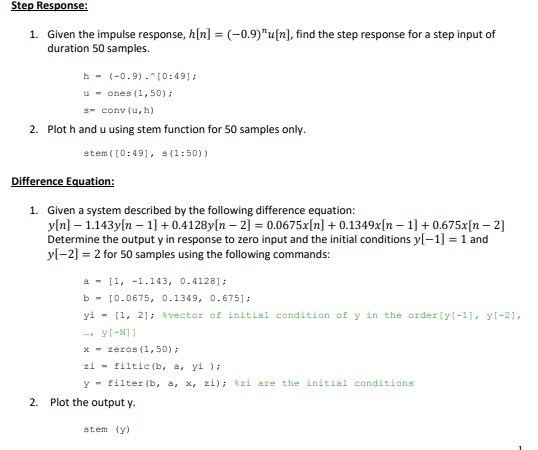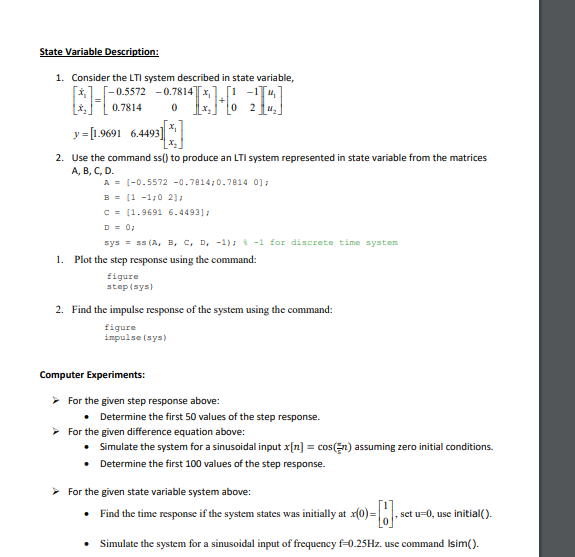1. Given the impulse response, h[n duration 50 samples. (-0.9)"u[n, find the step response for a step input of h-(0.9)-10:491 -ones (1,50) s- conv(u,h) 2. Plot h and u using stem function for 50 samples only stem(10:491, s(1:50) 1. Given a system described by the following difference equation: yIn] 1143yn 1 0.4128y[n -2 0.0675x[n0.1349xn 0.675x[n-2] Determine the output y in response to zero input and the initial conditionsy-11 and yl-2] 2 for 50 samples using the following commands: a -,-1.143, 0-41281 b-[0.0675, 0.1349, 0.6751 yi- [1, 2]; %vector of İnitial condition of y in the orderly-1), y[-2], x-zeros (1,50) zi " filtic (b, a, yi ); y- filter (b, a, x, zi); %zi are the initial conditions 2. Plot the output y. stem (y)
1. Consider the LTI system described in state variable, , 0.7814 0 2 y [.9691 64493 2. Use the command ss() to produce an LTI system represented in state variable from the matrices A, B, C, D [-0.5572-0.7814;0.7814 0]; A = B- -1:0 21a c = [1.9691 6.4493]; C, -1); -1 for discrete time system ss (A, B, D, sys 1. Plot the step response using the command: figure step(sys) 2. Find the impulse response of the system using the command: figure inpulse (sys For the given step response above: . Determine the first 50 values of the step response. For the given difference equation above: . Simulate the system for a sinusoidal input x[n] = cos( n) assuming zero initial conditions. . Determine the first 100 values of the step response. For the given state variable system above: Find the time response if the system states was inosct u-0, use initialO). Simulate the system for a sinusoidal input of frequency f-0.25Hz. use command Isim().

Step response:

clc;close all;clear all;
h=(-0.9).^[0:49];
u=ones(1,50)
s=conv(u,h)

subplot(311)
stem([0:49],u)
xlabel('n');ylabel('u(n)');title('step input')

subplot(312)
stem([0:49],h)
xlabel('n');ylabel('h(n)');title('Impulse response h(n)')

subplot(313)
stem([0:49],s(1:50))
xlabel('n');ylabel('s(n)');title('Step response s(n)')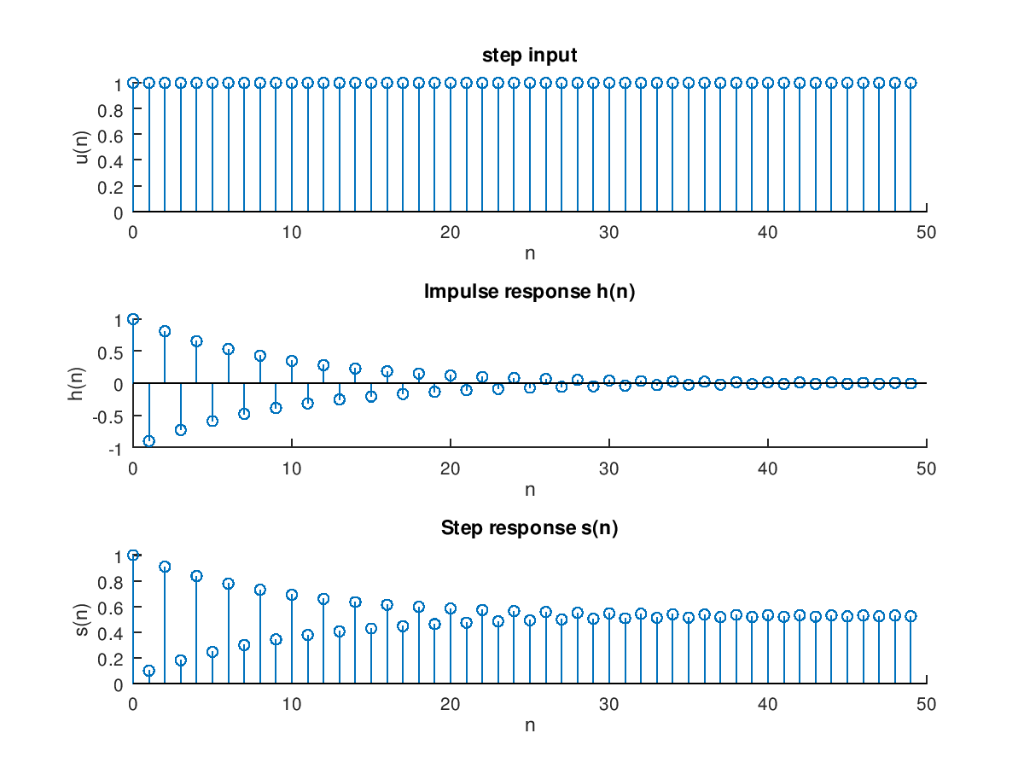Difference equation:

clc;close all;clear all;
a=[1 -1.143 0.4128]
b=[0.0675 0.1349 0.675]
%Zero input response
yi=[1 2]
x=zeros(1,50)
zi=filtic(b,a,yi)
y=filter(b,a,x,zi)
subplot(311)
stem([0:49],y(1:50))
xlabel('n');ylabel('y(n)');title('Zero input response')

%Zero state response
n=0:1:99
x=cos(pi*n/6)
y=filter(b,a,x)
subplot(312)
stem([0:99],y(1:100))
xlabel('n');ylabel('y(n)');title('Zero state response')

%Step response
x=ones(1,100)
y=filter(b,a,x)
subplot(313)
stem([0:99],y(1:100))
xlabel('n');ylabel('s(n)');title('Step response')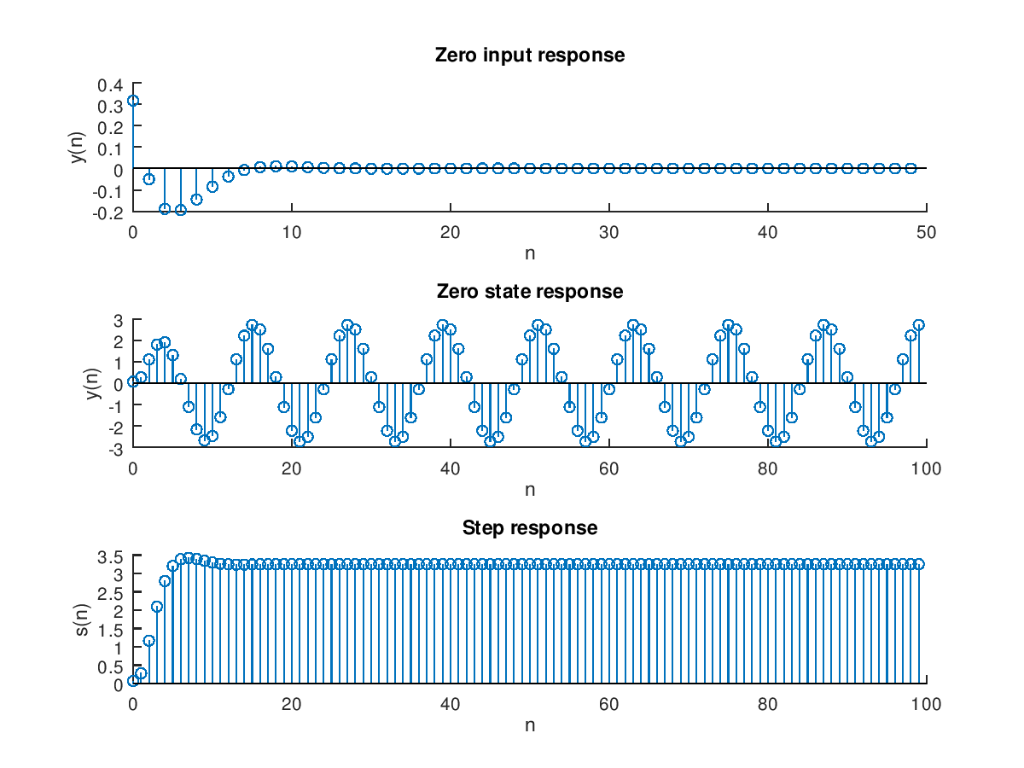State variable description:

clc;close all;clear all;
A=[-0.5572 -0/7814 ;0.7814 0];
B=[1 -1;0 2];
C=[1.9691 6.4493];
D=0;
sys=ss(A,B,C,D,0.1)
figure;
step(sys)

figure;
impulse(sys)

figure;
x0 = [1 ; 0];
initial(sys,x0)

figure;
%Response to sinusoidal input
f=0.25
[u,t] = gensig('sin',1/f,10,0.1);
lsim(sys,[u,u],t)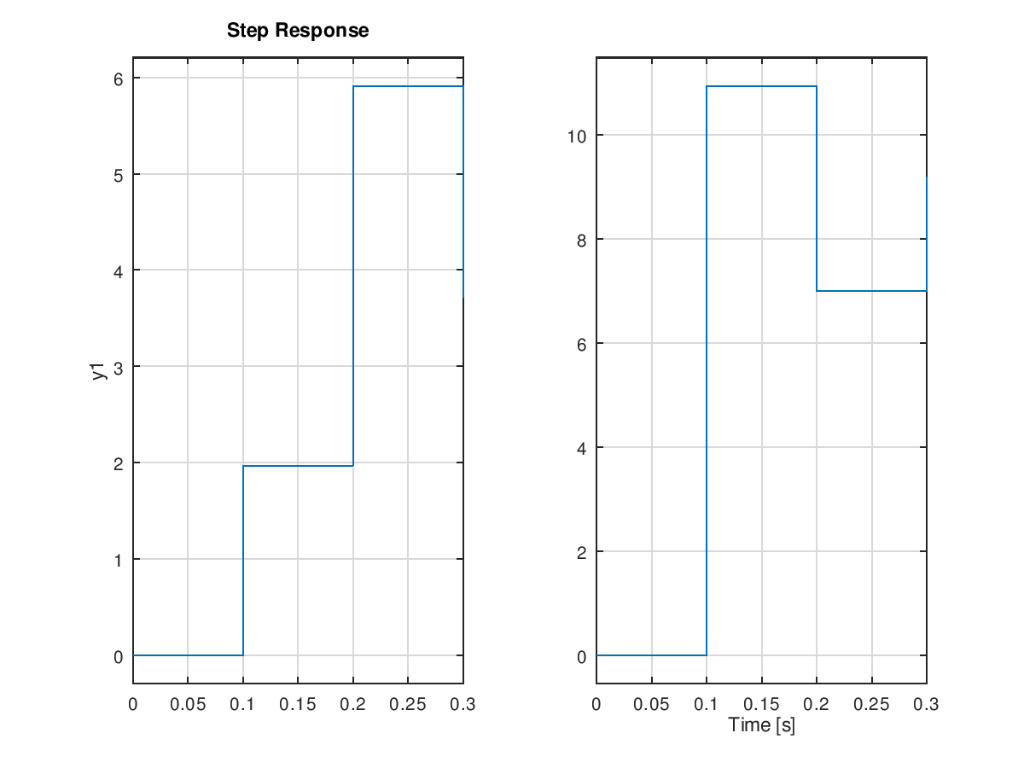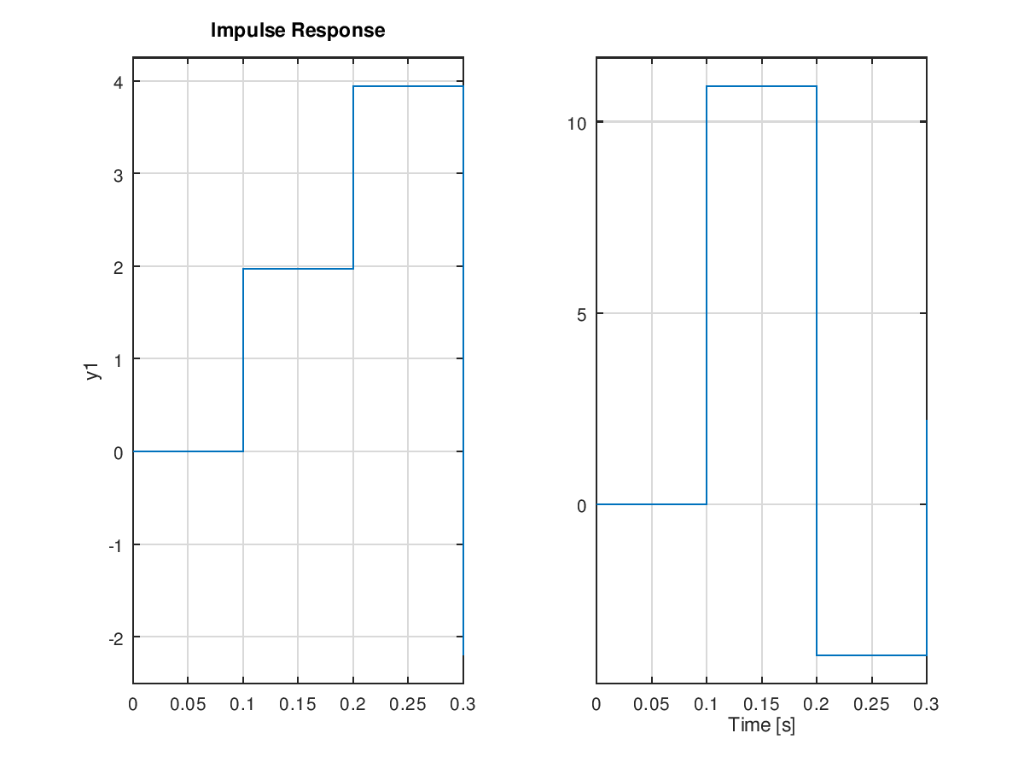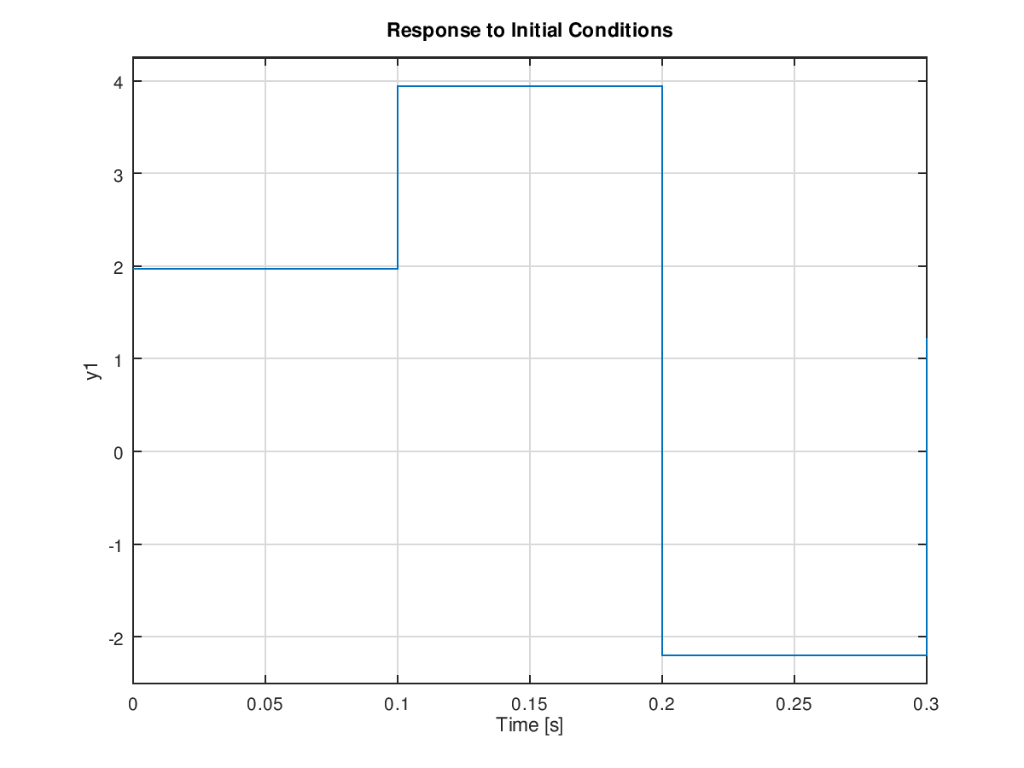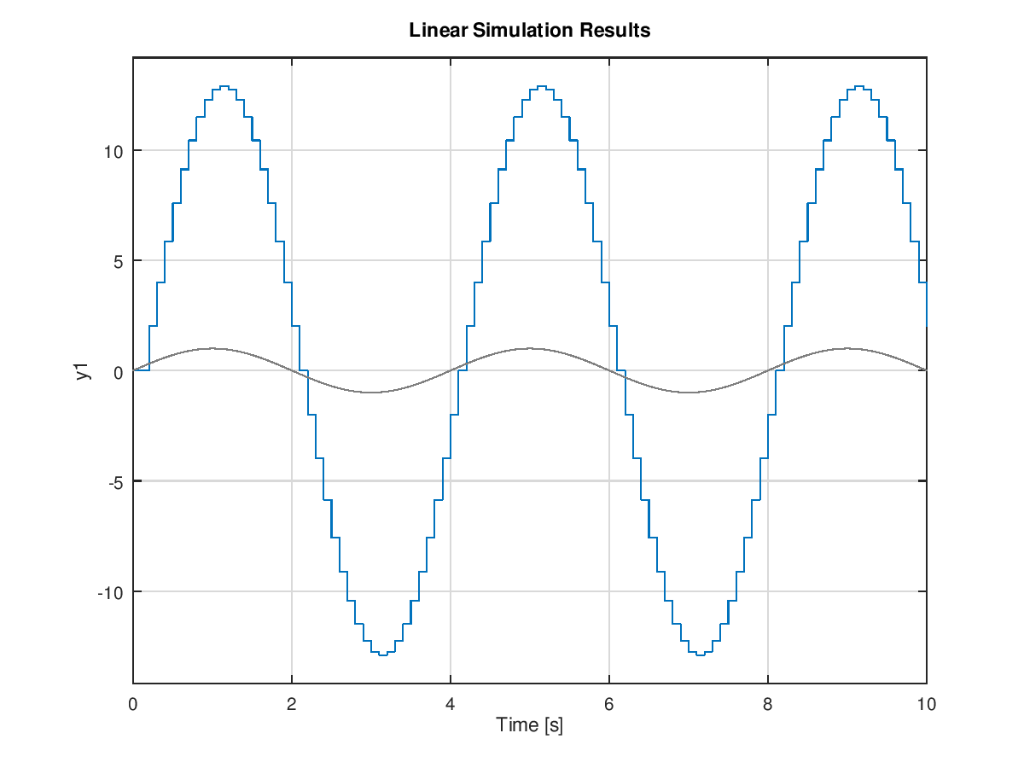##### Add Answer of: 1. Given the impulse response, h[n duration 50 samples. (-0.9)"u[n, find the step response for a step input of h-(0...
Similar Homework Help Questions
• ### (42)1+ (z-0.5)z-0.9)(z-0.8) 3. The transfer function of a system is H(z) = a) Compute an analytical expression for the...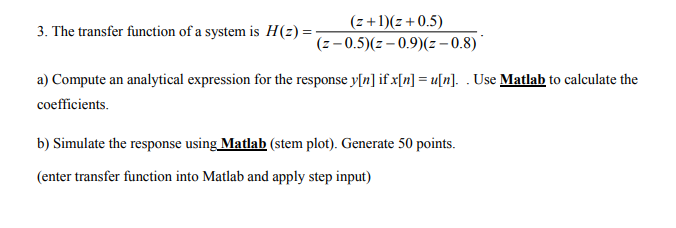(42)1+ (z-0.5)z-0.9)(z-0.8) 3. The transfer function of a system is H(z) = a) Compute an analytical expression for the response y[n] if x[n] = u[n]. . Use Matlab to calculate the coefficients b) Simulate the response using Matlab (stem plot). Generate 50 points. (enter transfer function into Matlab and apply step input) (42)1+ (z-0.5)z-0.9)(z-0.8) 3. The transfer function of a system is H(z) = a) Compute an analytical expression for the response y[n] if x[n] = u[n]. . Use Matlab...

• ### Problem 2: Find the impulse response h(n) of a causal LTI system if the input x(n)...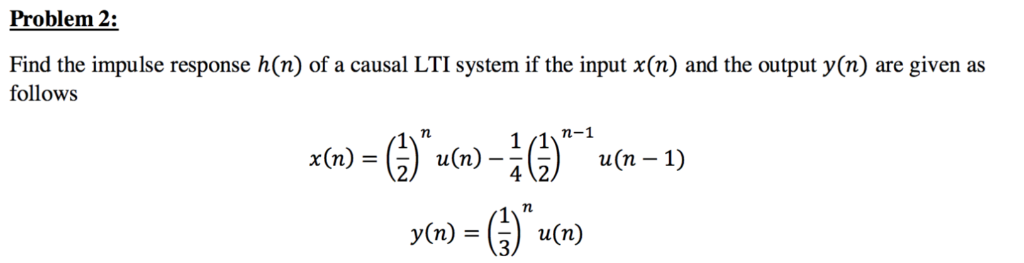Problem 2: Find the impulse response h(n) of a causal LTI system if the input x(n) and the output y(n) are given as follows 72 42)un-1) y(n)-G)na(n) xnun)

• ### do in matlab Question 2 (1 point) For the LTI system described by the following impulse response: h(n) n) n)n) 3) u(n 4) u Determine the following: 1) The system function representation, 2) The D...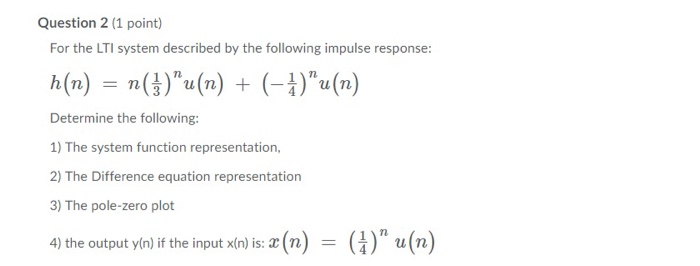do in matlab Question 2 (1 point) For the LTI system described by the following impulse response: h(n) n) n)n) 3) u(n 4) u Determine the following: 1) The system function representation, 2) The Difference equation representation 3) The pole-zero plot the output yin if the input xin)is(n) -()u(n) Question 2 (1 point) For the LTI system described by the following impulse response: h(n) n) n)n) 3) u(n 4) u Determine the following: 1) The system function representation, 2) The...

• ### The given input signal for 2.7.2 is:   x(t) = 3 cos(2 π t) + 6 sin(5 π t). Plz explain ...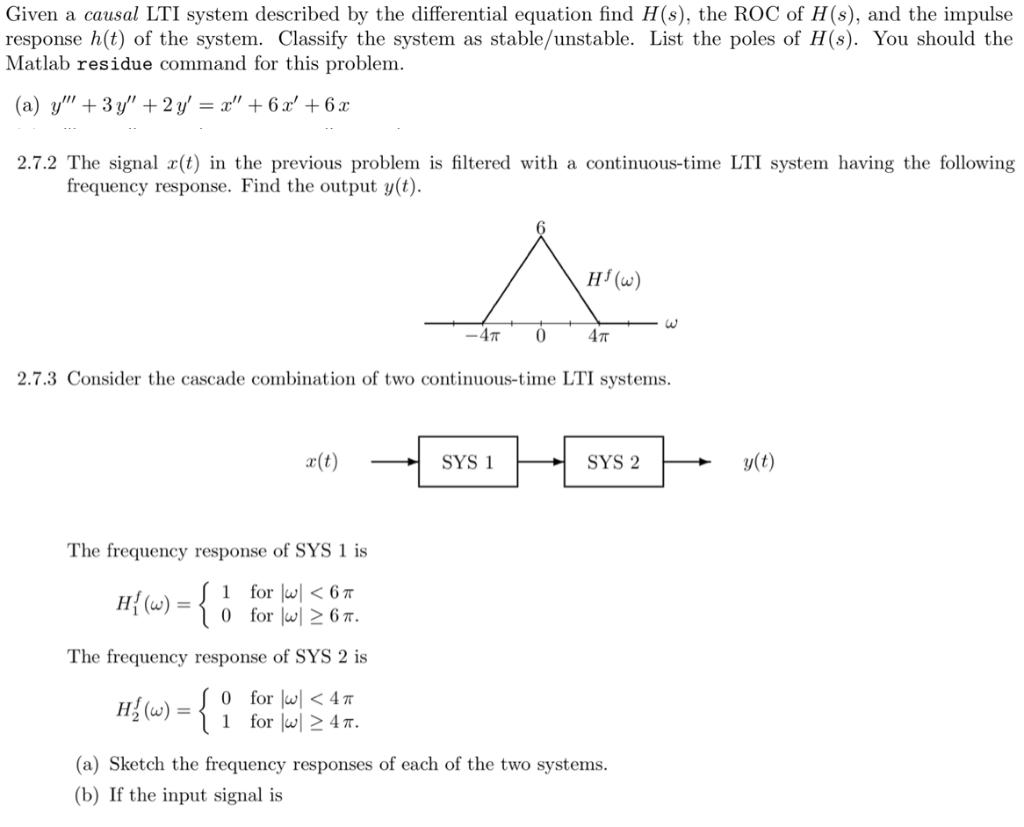The given input signal for 2.7.2 is:   x(t) = 3 cos(2 π t) + 6 sin(5 π t). Plz explain steps. Given a causal LTI system described by the differential equation find H(s), the ROC of H(s), and the impulse response h(t) of the system. Classify the system as stable/unstable. List the poles of H(s). You should the Matlab residue command for this problem 2.7.2 The signal x(t) in the previous problem is filtered with a continuous-time LTI system having...

• ### 2. A system is described by the following difference equation n]1.5y[n0.56y[n -2]+x{n-0.2x{n-] a) Find the transfer fun...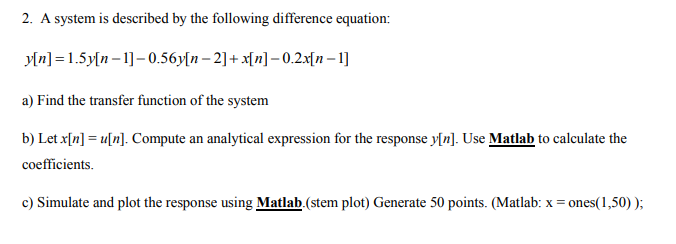2. A system is described by the following difference equation n]1.5y[n0.56y[n -2]+x{n-0.2x{n-] a) Find the transfer function of the system b) Let xn]un]. Compute an analytical expression for the response y[n]. Use Matlab to calculate the coefficients c) Simulate and plot the response using Matlab.(stem plot) Generate 50 points. (Matlab: x ones(1,50)); 2. A system is described by the following difference equation n]1.5y[n0.56y[n -2]+x{n-0.2x{n-] a) Find the transfer function of the system b) Let xn]un]. Compute an analytical expression for...

• ### A CT LTI system with impulse response h(t) = u(t)−u(t−1) is given the input x(t) = (1 − |t|)(u(t ...

A CT LTI system with impulse response h(t) = u(t)−u(t−1) is given the input x(t) = (1 − |t|)(u(t + 1) − u(t − 1)). Find the output of the system.

• ### (20 pts.) Determine the output sequence of the system with impulse response h[n] 6. u[n] when the input signal is x[n]...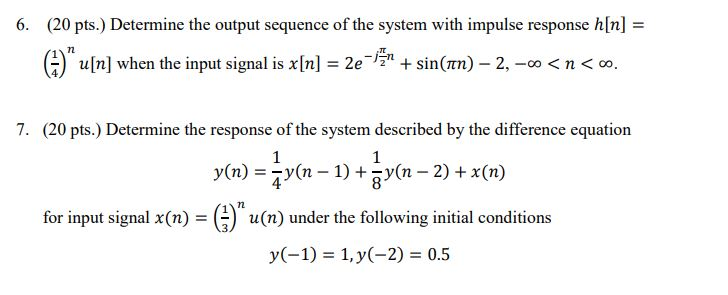(20 pts.) Determine the output sequence of the system with impulse response h[n] 6. u[n] when the input signal is x[n] = 2e-n + sin(nn)- 2, -co <n< 0o. 7. (20 pts.) Determine the response of the system described by the difference equation 1 1 y(n)y(n1)n2)x(n 8 7 for input signal x(n) u(n) under the following initial conditions 1, y(-2) 0.5 y(-1) (20 pts.) Determine the output sequence of the system with impulse response h[n] 6. u[n] when the input...

• ### Question 1 (10 pts): Consider the continuous-time LTI system S whose unit impulse response h is given by Le., h consists of a unit impulse at time 0 followed by a unit impulse at time (a) (2pts)...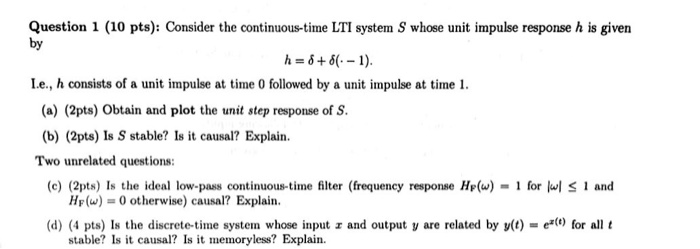Question 1 (10 pts): Consider the continuous-time LTI system S whose unit impulse response h is given by Le., h consists of a unit impulse at time 0 followed by a unit impulse at time (a) (2pts) Obtain and plot the unit step response of S. (b) (2pts) Is S stable? Is it causal? Explain Two unrelated questions (c) (2pts) Is the ideal low-pass continuous-time filter (frequency response H(w) for H()0 otherwise) causal? Explain (d) (4 pts) Is the discrete-time...

• ### 1) Given the system with u[n]- the discrete unit step input xiln 1] xiIn] r2ln] 0...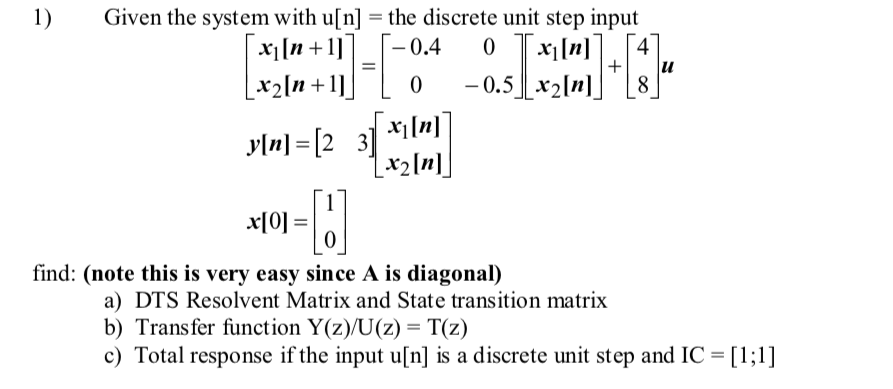1) Given the system with u[n]- the discrete unit step input xiln 1] xiIn] r2ln] 0 find: (note this is very easy since A is diagonal) a) DTS Resolvent Matrix and State transition matrix b) Transfer function Y(z)/U(z) = T(z) c) Total response if the input u[n] is a discrete unit step and IC [1;1]

• ### III.(6 pts.) A system is defined the following pole zero plot, where H(0)-10. a) Find the step response of the system.< Note: step response, not impulse response. b) (+3) Find the output, y()....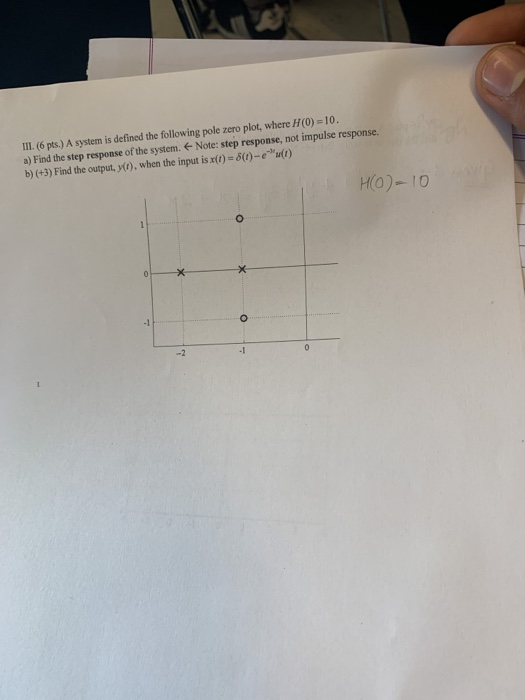III.(6 pts.) A system is defined the following pole zero plot, where H(0)-10. a) Find the step response of the system.< Note: step response, not impulse response. b) (+3) Find the output, y(). when the input is x()-8(0)-e) H(O) 10 -1 -2 III.(6 pts.) A system is defined the following pole zero plot, where H(0)-10. a) Find the step response of the system.

Need Online Homework Help?